# NCERT Solutions for Class 9 Maths Exercise 13.3## myCBSEguide App

CBSE, NCERT, JEE Main, NEET-UG, NDA, Exam Papers, Question Bank, NCERT Solutions, Exemplars, Revision Notes, Free Videos, MCQ Tests & more.

NCERT solutions for Class 9 Maths Surface Areas and Volumes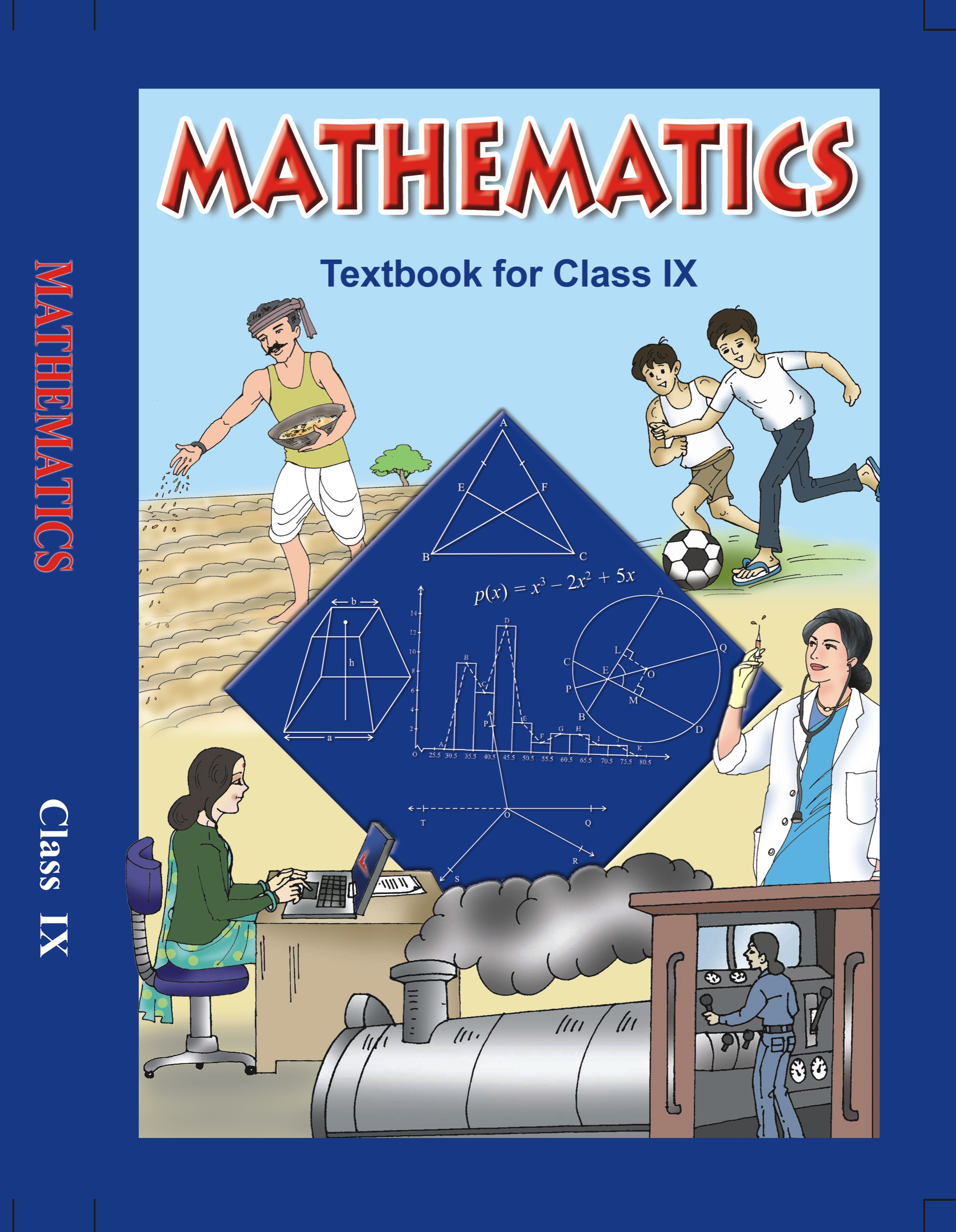## NCERT Solutions for Class 9 Mathematics Surface Areas and Volumes

###### 1. Diameter of the base of a cone is 10.5 cm and its slant height is 10 cm. Find its curved surface area and its total surface area.

Ans. Diameter = 10.5 cm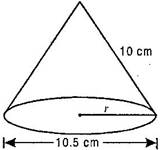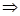Radius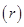=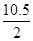=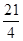cm

Slant height of cone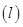= 10 cm

Curved surface area of cone=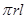=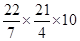= 165 cm2

Total surface area of cone =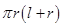=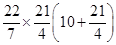=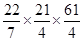= 251.625 cm2

###### 2. Find the total surface area of a cone, if its slant height is 21 cm and diameter of the base is 24 cm.

Ans. Slant height of cone= 21 m

Diameter of cone = 24 m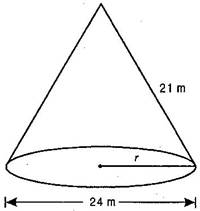Radius of cone=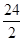= 12 m

Total surface area of cone ==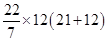=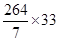= 1244.57 m2

###### 3. Curved surface area of a cone is 308 cm2 and its slant height is 14 cm. Find (i) radius of the base and  (ii) total surface area of the cone.

Ans. (i) Slant height of cone= 14 cm

Curved surface area of cone = 308 cm2= 308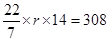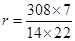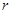= 7 cm

(ii) Total surface area of the cone

= Curved surface area + Area of circular base

=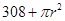=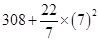= 462 cm2

NCERT Solutions for Class 9 Maths Exercise 13.3

###### 4. A conical tent is 10 m high and the radius of its base is 24 m. Find:

(i) slant height of the tent.

(ii) cost of the canvas required to make the tent, if the cost of a m2 canvas is Rs. 70.

Ans. Height of the conical tent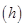= 10 m

Radius of the conical tent= 24 m

(i) Slant height of the tent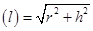=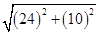=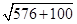=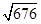= 26 m

(ii) Canvas required to make the tent

= Curved surface area of the tent==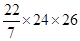=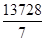m2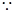Cost of 1 m2 canvas = Rs. 70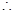Cost ofm2 canvas

= 70 x= Rs. 137280

NCERT Solutions for Class 9 Maths Exercise 13.3

###### 5. What length of tarpaulin 3 m wide will be required to make conical tent of height 8 m and base radius 6 m? Assume that the extra length of material that will be required for stitching margins and wastage in cutting is approximately 20 cm.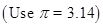Ans. Height of the conical tent= 8 m and Radius of the conical tent= 6 m

Slant height of the tent=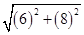=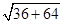=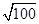= 10 m

Area of tarpaulin = Curved surface area of tent == 3.14 x 6 x 10 = 188.4 m2

Width of tarpaulin = 3 m

Let Length of tarpaulin = LArea of tarpaulin = Length x Breadth

= L x 3 = 3L

Now According to question,

3L = 188.4L =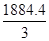= 62.8 m

The extra length of the material required for stitching margins and cutting is 20 cm = 0.2 m.

So the total length of tarpaulin bought is (62.8 + 0.2) m = 63 m

NCERT Solutions for Class 9 Maths Exercise 13.3

###### 6. The slant height and base diameter of a conical tomb are 25 m and 14 m respectively. Find the cost of whitewashing its curved surface at the rate of Rs. 210 per 100 m2.

Ans. Slant height of conical tomb= 25 m, Diameter of tomb = 14 mRadius of the tomb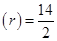= 7 m

Curved surface are of tomb ==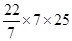= 550 m2Cost of white washing 100 m2

= Rs. 210Cost of white washing 1 m2 =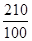Cost of white washing 550 m2

=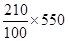= Rs. 1155

NCERT Solutions for Class 9 Maths Exercise 13.3

###### 7. A Joker’s cap is in the form of a right circular cone of base radius 7 cm and height 24 cm. Find the area of the sheet required to make 10 such caps.

Ans. Radius of cap= 7 cm,

Height of cap= 24 cm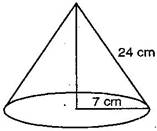Slant height of the cone=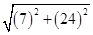=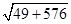=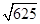= 25 cm

Area of sheet required to make a cap

= CSA of cone === 550 cm2Area of sheet required to make 10 caps = 10 x 550 = 5500 cm2

NCERT Solutions for Class 9 Maths Exercise 13.3

###### 8. A bus stop is barricaded from the remaining part of the road, by using 50 hollow cones made of recycled cardboard. Each cone has a base diameter of 40 cm and height 1 m. If the outer side of each of the cones is to be painted and the cost of painting is Rs. 12 per m2, what will be the cost of painting all these cones?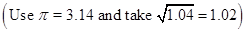Ans. Diameter of cone = 40 cmRadius of cone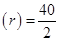= 20 cm

=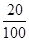m = 0.2 m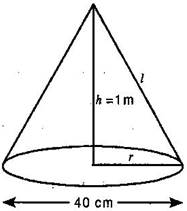Height of cone= 1 m

Slant height of cone=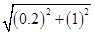=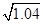m

Curved surface area of cone == 3.14 x 0.2 x= 0.64056 m2Cost of painting 1 m2 of a cone

= Rs. 12Cost of painting 0.64056 m2 of a cone

= 12 x 0.64056= Rs. 7.68672Cost of painting of 50 such cones

= 50 x 7.68672 = Rs. 384.34 (approx.)

## NCERT Solutions for Class 9 Maths Exercise 13.3

NCERT Solutions for Class 9 Maths PDF (Download) Free from myCBSEguide app and myCBSEguide website. Ncert solution class 9 Maths includes text book solutions from Mathematics Book. NCERT Solutions for CBSE Class 9 Maths have total 15 chapters. 9 Maths NCERT Solutions in PDF for free Download on our website. Ncert Maths class 9 solutions PDF and Maths ncert class 9 PDF solutions with latest modifications and as per the latest CBSE syllabus are only available in myCBSEguide.

## CBSE app for Class 9

To download NCERT Solutions for Class 9 Maths, Computer Science, Home Science,Hindi ,English, Social Science do check myCBSEguide app or website. myCBSEguide provides sample papers with solution, test papers for chapter-wise practice, NCERT solutions, NCERT Exemplar solutions, quick revision notes for ready reference, CBSE guess papers and CBSE important question papers. Sample Paper all are made available through the best app for CBSE students and myCBSEguide website.### 5 thoughts on “NCERT Solutions for Class 9 Maths Exercise 13.3”

1. Thank u so much becoz of u I understood the whole exercise

2. thank you

3. thanks you can help me

4. Very good answer in maths solutions , I am proud of you mycbseguide. Com

5. Very good solution for class 9 in maths / thank you